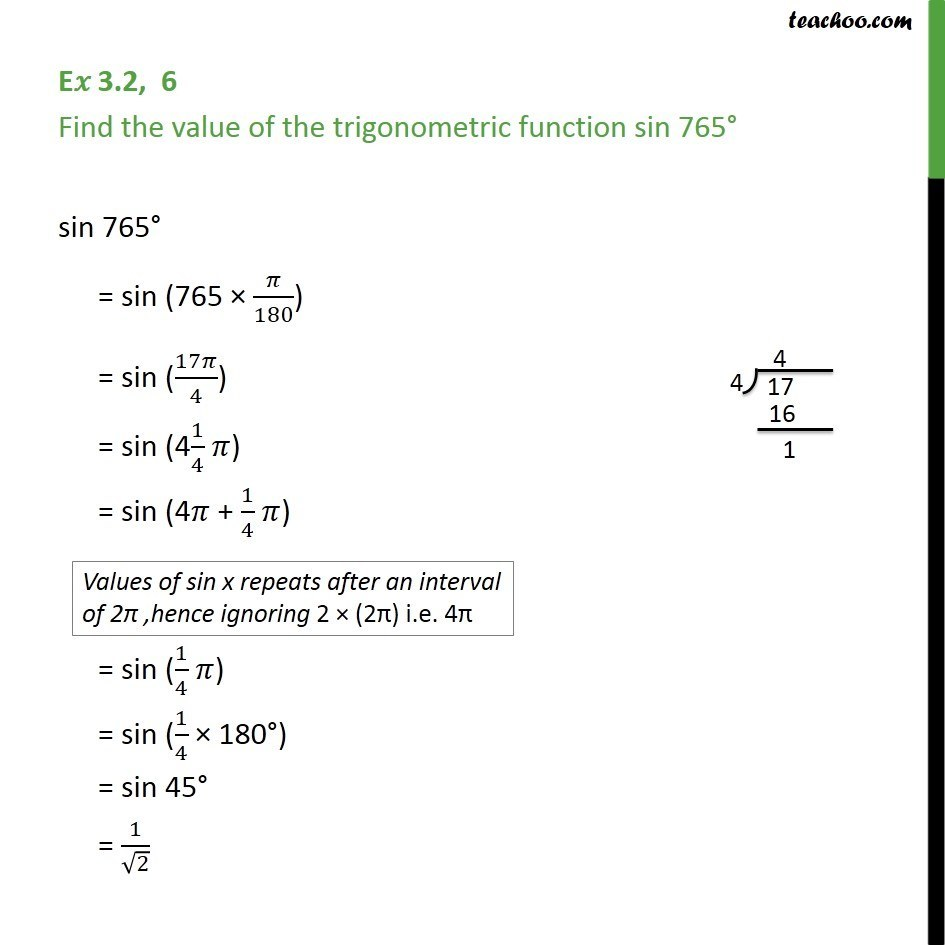Learn All Concepts of Chapter 2 Class 11 Relations and Function - FREE. Check - Trigonometry Class 11 - All Concepts1. Chapter 3 Class 11 Trigonometric Functions
2. Concept wise
3. Finding Value of trignometric functions, given angle

Transcript

E𝑥 3.2, 6 Find the value of the trigonometric function sin 765° sin 765° = sin (765 × 𝜋/180) = sin (17𝜋/4) = sin (41/4 𝜋) = sin (4𝜋 + 1/4 𝜋) = sin (1/4 𝜋) = sin (1/4 × 180°) = sin 45° = 1/√2

Finding Value of trignometric functions, given angle

About the AuthorDavneet Singh
Davneet Singh is a graduate from Indian Institute of Technology, Kanpur. He has been teaching from the past 9 years. He provides courses for Maths and Science at Teachoo.